International
Tables for
Crystallography
Volume B
Reciprocal space
Edited by U. Shmueli

International Tables for Crystallography (2006). Vol. B, ch. 5.1, pp. 549-550   | 1 | 2 |

## Section 5.1.8.3. Spherical-wave Pendellösung

A. Authiera*

aLaboratoire de Minéralogie-Cristallographie, Université P. et M. Curie, 4 Place Jussieu, F-75252 Paris CEDEX 05, France
Correspondence e-mail: authier@lmcp.jussieu.fr

#### 5.1.8.3. Spherical-wave Pendellösung

| top | pdf |

Fig. 5.1.8.4shows that along any path Ap inside the Borrmann triangle two wavefields propagate, one with tie point, on branch 1, the other with the point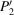, on branch 2. These two points lie on the extremities of a diameter of the dispersion surface. The two wavefields interfere, giving rise to Pendellösung fringes, which were first observed by Kato & Lang (1959), and calculated by Kato (1961b). These fringes are of course quite different from the plane-wave Pendellösung fringes predicted by Ewald (Section 5.1.6.3)because the tie points of the interfering wavefields are different and their period is also different, but they have in common the fact that they result from interference between wavefields belonging to different branches of the dispersion surface.Figure 5.1.8.4 | top | pdf |Interference at the origin of the Pendellösung fringes in the case of an incident spherical wave. (a) Direct space; (b) reciprocal space.

Kato has shown that the intensity distribution at any point at the base of the Borrmann triangle is proportional to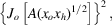where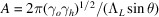and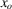and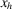are the distances of p from the sides AB and AC of the Borrmann triangle (Fig. 5.1.8.4). The equal-intensity fringes are therefore located along the locus of the points in the triangle for which the product of the distances to the sides is constant, that is hyperbolas having AB and AC as asymptotes (Fig. 5.1.8.4b). These fringes can be observed on a section topograph of a wedge-shaped crystal (Fig. 5.1.8.5). The technique of section topography is described in IT C, Section 2.7.2.2. The Pendellösung distance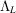depends on the polarization state [see equation (5.1.3.8)]. If the incident wave is unpolarized, one observes the superposition of the Pendellösung fringes corresponding to the two states of polarization, parallel and perpendicular to the plane of incidence. This results in a beat effect, which is clearly visible in Fig. 5.1.8.5.Figure 5.1.8.5 | top | pdf |Spherical-wave Pendellösung fringes observed on a wedge-shaped crystal. (a) Computer simulation (solid lines: maxima; dashed lines: minima). (b) X-ray section topograph of a wedge-shaped silicon crystal (444 reflection, Mo Kα radiation).

### References

Kato, N. (1961b). A theoretical study of Pendellösung fringes. Part 2. Detailed discussion based upon a spherical wave theory. Acta Cryst. 14, 627–636.Google Scholar
Kato, N. & Lang, A. R. (1959). A study of Pendellösung fringes in X-ray diffraction. Acta Cryst. 12, 787–794.Google Scholar